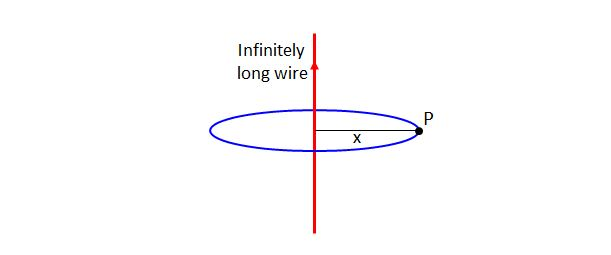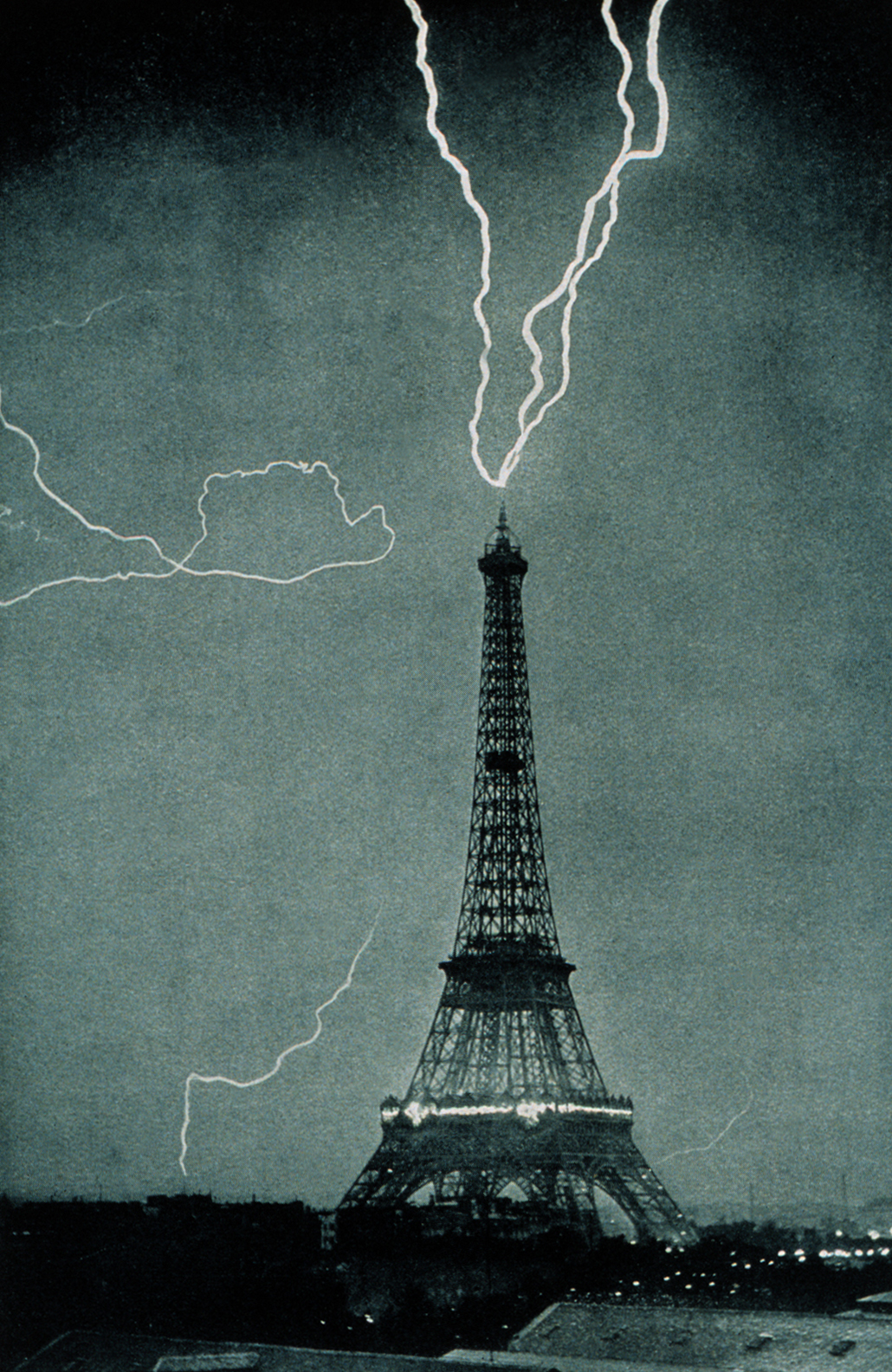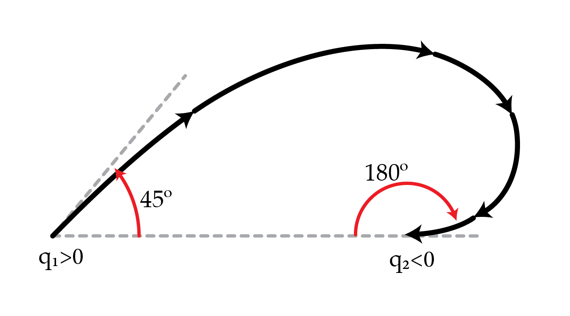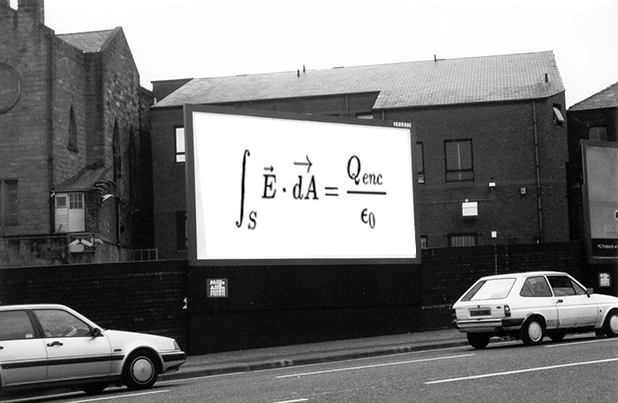Electricity and Magnetism

# Electric Flux: Level 2-3 ChallengesSuppose that an infinitely long straight current-carrying wire has a uniform linear charge density $\lambda.$ Let this wire be on the $y$-axis of the $xy$-plane, and let $x > 0$ be the distance between the $y$-axis and a point $P$ on the $xy$-plane. What is the strength of the electric field at the point $P?$

Note: $\epsilon_0$ in the choices below denotes the electric constant.This lightning strike provides evidence thatGauss's law is a very powerful method to determine the electric field due to a distribution of charges. The mathematical expression for Gauss's law is

$\int_{S} \vec{E} \cdot \vec{dA}=\frac{Q_{enc}}{\epsilon_0} ,$

where $S$ is a surface, $\vec{E}$ is the electric field vector, $\vec{dA}$ is the infinitesimal area element, $Q_{enc}$ is the charge enclosed by $S,$ and $\epsilon_0$ is a constant.

In order to apply Gauss's law, we need to understand what each of the parts of this expression means. This set of problems will help you understand each of the components. Let's start with $S$. You may be more familiar with integrals as the limit of a sum of a function over a line interval, which gives the "area under the curve." An integral over a surface of a function is just the sum of that function over all the points on the surface.

The surface in Gauss's law is a closed two-dimensional surface, such as the surface of a sphere or the surface of a cube. A closed surface is a surface that divides space into an inside and an outside, whereby dividing we mean there is no path that goes from inside to outside that does not penetrate the surface. Consider the surface $S$ of the objects below. For which of the objects is $S$ a closed surface?Consider two charges $q_{1}>0$ and $q_{2}<0$. An electric field line exits the positive charge $q_{1}$ at $45$ degrees and enters the negative charge $q_2$ at $180$ degrees. What is the ratio $\frac{|q_{1}|}{|q_{2}|}$?Gauss' law is a very powerful method to determine the electric field due to a distribution of charges. The mathematical expression for Gauss' law is:

$\int_{S} \vec{E} \cdot \vec{dA}=\frac{Q_{enc}}{\epsilon_0}$

where $S$ is a surface, $\vec{E}$ is the electric field vector, $\vec{dA}$ is the infinitesimal area element, $Q_{enc}$ is the charge enclosed by $S$ and $\epsilon_0$ is a constant.

We now turn to the notion of integration over a specified surface. One can think about definite integration over a line interval, i.e. $\int_a^b f(x) dx$, as the area under the curve defined by $f(x)$. Similarly, the integral over a surface of a function can be thought of as the volume under the 2-d graph of the function. Using this definition, what is the integral

$\int_S x dA$

where the surface $S$ is the square in the $xy$-plane with corners at $(0,0), (1,0),(0,1),(1,1)$.

This problem is part of David's set on Gauss' Law.

×